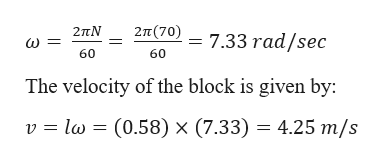# A 300 g block on a 58-cm-long string swings in a circle on a horizontal, frictionless table at 70 rpm.What is the speed of the block?What is the tension in the string?

Question

A 300 g block on a 58-cm-long string swings in a circle on a horizontal, frictionless table at 70 rpm.

What is the speed of the block?

What is the tension in the string?

check_circleExpert Solution
Step 1

Given information:

Mass of the block (m) = 300 g = 0.3 kg

Length of the string (l) = 58 cm = 0.58 m

Revolution per minute (N) = 70 rpm

Step 2

We know that the angular velocity of the block is given by:help_outlineImage Transcriptionclose2π (70) 2πΝ 7.33 rad/sec 60 60 The velocity of the block is given by: l (0.58) x (7.33) = 4.25 m/s v fullscreen
Step 3

The tension in the string is equal to the c...

### Want to see the full answer?

See Solution

#### Want to see this answer and more?

Solutions are written by subject experts who are available 24/7. Questions are typically answered within 1 hour*

See Solution
*Response times may vary by subject and question
Tagged in

### Physics